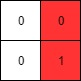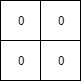##### Welcome to Subscribe On Youtube

Formatted question description: https://leetcode.ca/all/1428.html

# 1428. Leftmost Column with at Least a One (Medium)

(This problem is an interactive problem.)

A binary matrix means that all elements are 0 or 1. For each individual row of the matrix, this row is sorted in non-decreasing order.

Given a row-sorted binary matrix binaryMatrix, return leftmost column index(0-indexed) with at least a 1 in it. If such index doesn't exist, return -1.

You can't access the Binary Matrix directly.  You may only access the matrix using a BinaryMatrix interface:

• BinaryMatrix.get(x, y) returns the element of the matrix at index (x, y) (0-indexed).
• BinaryMatrix.dimensions() returns a list of 2 elements [m, n], which means the matrix is m * n.

Submissions making more than 1000 calls to BinaryMatrix.get will be judged Wrong Answer.  Also, any solutions that attempt to circumvent the judge will result in disqualification.

For custom testing purposes you're given the binary matrix mat as input in the following four examples. You will not have access the binary matrix directly.

Example 1:Input: mat = [[0,0],[1,1]]
Output: 0


Example 2:Input: mat = [[0,0],[0,1]]
Output: 1


Example 3:Input: mat = [[0,0],[0,0]]
Output: -1

Example 4:Input: mat = [[0,0,0,1],[0,0,1,1],[0,1,1,1]]
Output: 1


Constraints:

• m == mat.length
• n == mat[i].length
• 1 <= m, n <= 100
• mat[i][j] is either 0 or 1.
• mat[i] is sorted in a non-decreasing way.
// OJ: https://leetcode.com/problems/leftmost-column-with-at-least-a-one/
// Time: O(M * logN)
// Space: O(1)
class Solution {
int search(BinaryMatrix &A, int i, int end) {
int L = 0, R = end - 1;
while (L <= R) {
int M = (L + R) / 2, val = A.get(i, M);
if (val == 0) L = M + 1;
else if (val == 1) {
if (M - 1 < 0 || A.get(i, M - 1) == 0) return M;
R = M - 1;
}
}
return -1;
}
public:
int leftMostColumnWithOne(BinaryMatrix &A) {
auto dim = A.dimensions();
int M = dim, N = dim, ans = N;
for (int i = 0; i < M; ++i) {
int j = search(A, i, ans);
if (j != -1) ans = j;
}
return ans == N ? -1 : ans;
}
};

• /**
* // This is the BinaryMatrix's API interface.
* // You should not implement it, or speculate about its implementation
* interface BinaryMatrix {
*     public int get(int row, int col) {}
*     public List<Integer> dimensions {}
* };
*/

class Solution {
public int leftMostColumnWithOne(BinaryMatrix binaryMatrix) {
List<Integer> dimensions = binaryMatrix.dimensions();
int rows = dimensions.get(0), columns = dimensions.get(1);
int leftMostColumn = columns;
for (int i = 0; i < rows; i++) {
int low = 0, high = leftMostColumn - 1;
if (binaryMatrix.get(i, high) == 0)
continue;
leftMostColumn = Math.min(leftMostColumn, high);
while (low < high) {
int mid = (high - low) / 2 + low;
int num = binaryMatrix.get(i, mid);
if (num == 1) {
high = mid;
leftMostColumn = Math.min(leftMostColumn, mid);
} else
low = mid + 1;
}
if (leftMostColumn == 0)
break;
}
return leftMostColumn == columns ? -1 : leftMostColumn;
}
}

############

/**
* // This is the BinaryMatrix's API interface.
* // You should not implement it, or speculate about its implementation
* interface BinaryMatrix {
*     public int get(int row, int col) {}
*     public List<Integer> dimensions {}
* };
*/

class Solution {
public int leftMostColumnWithOne(BinaryMatrix binaryMatrix) {
List<Integer> scale = binaryMatrix.dimensions();
int rows = scale.get(0), cols = scale.get(1);
int res = -1;
for (int row = 0; row < rows; ++row) {
int left = 0, right = cols - 1;
while (left < right) {
int mid = (left + right) >> 1;
if (binaryMatrix.get(row, mid) == 1) {
right = mid;
} else {
left = mid + 1;
}
}
if (binaryMatrix.get(row, left) == 1) {
if (res == -1) {
res = left;
} else {
res = Math.min(res, left);
}
}
}
return res;
}
}

• // OJ: https://leetcode.com/problems/leftmost-column-with-at-least-a-one/
// Time: O(M * logN)
// Space: O(1)
class Solution {
int search(BinaryMatrix &A, int i, int end) {
int L = 0, R = end - 1;
while (L <= R) {
int M = (L + R) / 2, val = A.get(i, M);
if (val == 0) L = M + 1;
else if (val == 1) {
if (M - 1 < 0 || A.get(i, M - 1) == 0) return M;
R = M - 1;
}
}
return -1;
}
public:
int leftMostColumnWithOne(BinaryMatrix &A) {
auto dim = A.dimensions();
int M = dim, N = dim, ans = N;
for (int i = 0; i < M; ++i) {
int j = search(A, i, ans);
if (j != -1) ans = j;
}
return ans == N ? -1 : ans;
}
};

• # """
# This is BinaryMatrix's API interface.
# You should not implement it, or speculate about its implementation
# """
# class BinaryMatrix(object):
#    def get(self, row: int, col: int) -> int:
#    def dimensions(self) -> list[]:

class Solution:
def leftMostColumnWithOne(self, binaryMatrix: 'BinaryMatrix') -> int:
rows, cols = binaryMatrix.dimensions()
res = -1
for row in range(rows):
left, right = 0, cols - 1
while left < right:
mid = (left + right) >> 1
if binaryMatrix.get(row, mid) == 1:
right = mid
else:
left = mid + 1
if binaryMatrix.get(row, left) == 1:
if res == -1:
res = left
else:
res = min(res, left)
return res


• /**
* // This is the BinaryMatrix's API interface.
* // You should not implement it, or speculate about its implementation
* type BinaryMatrix struct {
*     Get func(int, int) int
*     Dimensions func() []int
* }
*/

func leftMostColumnWithOne(binaryMatrix BinaryMatrix) int {
scale := binaryMatrix.Dimensions()
rows, cols := scale, scale
res := -1
for row := 0; row < rows; row++ {
left, right := 0, cols-1
for left < right {
mid := (left + right) >> 1
if binaryMatrix.Get(row, mid) == 1 {
right = mid
} else {
left = mid + 1
}
}
if binaryMatrix.Get(row, left) == 1 {
if res == -1 {
res = left
} else {
res = min(res, left)
}
}
}
return res
}

func min(a, b int) int {
if a < b {
return a
}
return b
}